American Journal of Civil Engineering
Volume 3, Issue 5, September 2015, Pages: 140-149

Thermo-Physical Properties of Local Materials Used in the Construction of Chad

Ahmat-Charfadine Mahamat1, Mahamat Barka1, Abakar Mahamat Tahir1, Malloum Soultan1,
Salif Gaye2, Aboubakar Cheikh Beye2

1Laboratoire des Energies Renouvelables et des Matériaux Locaux de Faculté des Sciences Exactes et Appliquées de l’Université de N’Djaména, Tchad

2Laboratoire de Matériaux, Mécanique et Hydraulique de la Faculté des Sciences et Techniques de l’Université de Thiès, Sénégal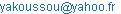(Ahmat-Charfadine M.)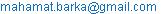(M. Barka)(Abakar. M. T.)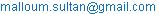(M. Soultan)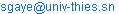(S. Gaye)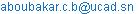(A. C. Beye)

Ahmat-Charfadine Mahamat, Mahamat Barka, Abakar Mahamat Tahir, Malloum Soultan, Salif Gaye, Aboubakar Cheikh Beye. Thermo-Physical Properties of Local Materials used in the Construction of Chad. American Journal of Civil Engineering. Vol. 3, No. 5, 2015, pp. 140-149. doi: 10.11648/j.ajce.20150305.12

Abstract: As earth is the most commonly used construction material in Chad, the purpose of the present study is to characterize it as a whole, and to characterize its thermo physical properties. Various tests were conducted on it and mainly on its thermo-physical characteristics, as these significantly affect the comfort of a house. The study focused on earth taken from a climate zone of Chad, mixed with straw at a given proportion or used alone. Different values of thermal conductivity and diffusivity of these materials as well as their variations in relation with water continence are presented.

Keywords: Construction Materials, Earth/Clay, Thermo-Physical Characteristics, Thermal Conductivity, Thermal Diffusivity, Housing

1. Introduction

Nowadays in rural or urban Sahel which Chad is a part, various materials are used for the construction of housing. These materials range from earth mixed with straw commonly called "banco", to the mixture of cement and sand or even concrete. The purpose of this research is the study of thermo-physical properties of the material earth used in the construction of housing in Chad. Given the diversity of these materials in either parts of the country, it is important to model the thermo-physical behavior of this type of construction material. As for the materials considered as porous, there are many possible models at our disposal.

Assuming that earth shaped as bricks is treated as a porous material; we selected the parallel series configuration with a distribution q when the pores are filled with air. We present the different testing methods used to study the thermo-physical properties of the material under consideration and the test results conducted on the different materials studied on the one hand, and we carried out the practical study of thermal modeling of these materials on the other.

2. Studied Materials

Raw earth, as used in building construction since the earliest times and evidenced by traditional housing in numerous parts of the planet, is the subject of our study. As it is a highly heterogeneous material with very different characteristics from one region to another, as well as being a very large resource, its precise knowledge before any use in construction is important. Numerous laboratory tests and in situ tests can be performed to identify and characterize the thermal conditions of a soil. In order to establish a reliable diagnosis for the use of earth in construction, a number of these tests are absolutely necessary.

2.1. Preparation of Samples

2.1.1. Choice of Site

Given the very large size of the country and taking into account the diversity of its climate zones and meteorological data, we limited our study to the earth materials used in the city of N'Djamena, located in the Sahelian zone. The earth studied is one taken from quarries closest to the above-mentioned city and from extraction depths comprised between 1.50 and 2.50 meters. On that soil, we carried out a few determination tests on its thermo-physical properties and assessed its potential for use in construction as well as its contribution to thermal comfort.

2.1.2. Production of Samples

The making of earth samples derives from the traditional technique of making mud bricks , , . A certain amount of earth is taken from the collected material, poured into a tank, and a certain amount of water is added. The whole is mixed until a consistency closer to plasticity is reached or even exceeded, generally. The paste is then packed into a mold designed for this purpose until completely filled. The molded mixture is left for 24 hours at room temperature in the laboratory.

After 24 hours of drying, that mixture is placed in an oven at a temperature of 105 °C ± 5 °C for 24 hours. After drying, the samples are left to cool for 15 to 20 minutes before the last weighing. In order to obtain baked bricks, the samples are made, dried, and left to bake in an oven at a temperature of 300 ° C to 400 ° C for twelve hours. To measure the thermo physical characteristics of the material by the method known as "method of boxes" presented in Chapter 2, we made samples of adobe (earth + straw) destined to be specifically placed in the measuring cell. Table 1show the dimensions and detailed compositions of samples made and dried in an oven for 24 hours.

Table 1. Dimensions and compositions of the samples.

 Designation N’Djamena Composition Earth alone Earth and straw Mass of earth (kg) 3,5 0,5 Mass of straw (kg) 0 0,05 Volume (cm3) 23,28 x 22,80 x 2,54 17,52 x 8,29 x 1,84 Mass (dry) in kg 2,786 0,409 a (kg/m3) 2068 1529

3. Measurement Methodology and Description of the Material

Conductivity, diffusivity, mass-related heat and thermal resistance are the most important features to consider when choosing a heat exchanger material. It is important to remember that conductivity is measured in steady state while diffusivity is involved in transient state and, in most cases these two quantities are the measured ones. The two others are derived by computation. Many methods are used to measure conductivity and thermal diffusivity. We used the Boxes Method developed in the Laboratory of Solar and Thermal Studies of Claude Bernard University. It is relatively fast, of a simple handling technique, and has a level of precision comparable to others. This method has been the subject of several publications , , , , ; It uses samples of significant sizes, and the associated measurements are made in the actual conditions of use of the materials. Figure 1 provides an overview of the experimental device the main elements of which are described below: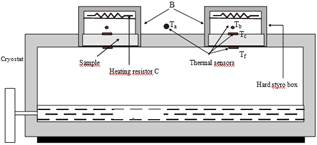Figure 1. Sectional view of the cold capacitor and hot boxes.

The measuring cell consists of:

A lower chamber of size 2m x 1m x 0.45m that is highly insulated and kept at low temperature (up to-10 ° C). It is supplied with brine cooler. This cooler that we have named "exchanger" is powered by a HAAKE circulating bath cryostat of type DC1.

Two identical plywood boxes B isolated from the inside by the styrodur and each equipped with a heat emitter C allow two simultaneous measurements of conductivity and diffusivity.

Ÿ In order to measure the level of conductivity, the box is coated on the inside of its upper surface with a film of low power (<10 W) heating element in Zegadi , , whose heat output is controlled with a rheostat.

Ÿ For the measurement of diffusivity, the box is equipped with a high power incandescent lamp of 500 to 1500 W.

All the wiring connections of the temperature sensors and film heating power source are connected to a box that can in turn be connected either to a measurement console or a type E 7100 hybrid central data acquisition recorder with 32 inlets and equipped with an RS232C communication interface. It allows the use of a 286 PC compatible remote computer to access the information programmed in Turbo Pascal 6.0 language for storage or modification, and obtains measurement results automatically, among others.

Temperature sensors that are surface and ambient atmosphere probes divided between the two faces of the sample that measures the temperature of the hot surface (Tc) and that of the cold surface (Tf) as well as within the box that measures the ambient temperature(Tb). Another sensor common to both boxes measures the ambient temperature of the room (Ta). The samples used have parallelepiped shapes and 3 to 7 cm thick. Their square sections have 27 cm sides.

3.1. Measurement of Thermal Conductivity

3.1.1. Evaluation of Thermal Conductivity

Conductivity is a function of temperature. The expression of conductivity averaged over the interval [Tc, Tf] is given by(1.1)

Thus, lm is the conductivity corresponding to the average temperature

Tm = (Tc + Tf) / 2 for a temperature differenceD T = Tc-Tf.

This correspondence between lm and Tm requires linear dependence between the two measurements. In steady state, the heat flow that goes through the sample is written as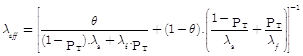with: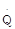: Power emitted by the resistance formulated by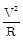(where V is the voltage across resistor R)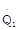: Power exchanged between the inside and outside of the box (it is formulated by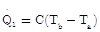, C is the coefficient of heat loss through the box and is expressed in W / °C. It is either determined experimentally or calculated).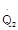: Thermal power passing through the sample. It is formulated by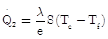where e represents the thickness of the sample, S the useful area also known as measurement area and slightly less than (27 x 27) cm2 in size. In our calculations, we used the area described as corrected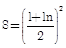instead, taking into account the very geometry of the box (Figure 1.2).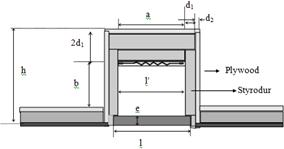Figure 2. Cross section of a measuring box.

In order to calculate C, we can use the formula given by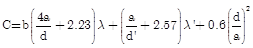(1.2)

With d = d1 + d2 and d '= 2d1 + d2;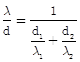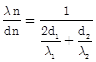l1: thermal conductivity of the hard styro that equals 0,033 W.m-1 styrodur-1.K-1

l2: thermal conductivity of the plywood that equals 0,12 W.m-1plated.K-1.

In order to minimize the lateral losses and to determine conductivity with good accuracy, the emission of heat inside the box is adjusted in such a way that Tb becomes slightly greater than Ta and Tb - Ta< 1°C.

Using the heat balance expressed above, the value of the effective thermal conductivity is obtained in continuous state by: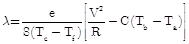(1.3)

Errors corresponding to the test results are systematic errors and random errors. The calculation of this error resulted in: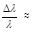5%. It can be said that the relative error in the determination of continuous thermal conductivity by the method of box is about 5%.

3.1.2. Measurements

a)  Measurement of Tb - Ta

An ambient heat sensor is placed near the boxes and measures the room temperature Ta. Another one that measures Tb, is placed inside the box in a suitable position (middle), so that the temperature is averaged throughout the volume. In the determination of Tb - Ta, and up to the measurements checking, one must assure that the outer atmosphere had been stable for at least two hours. Indeed, because of the high inertia of the box, a change in temperature of the room is not transmitted immediately inside the box.

b)  Measurement of Tc-Tf

Two contact probes are distributed on both sides of the sample. On the cold side, the temperature is strictly uniform (homogeneous). However, on the warm side, the edges of the sample are slightly colder than the center. The difference being constant, a systematic correction is made.

c)    Measurement of V and of R

Heat is generated by Joule effect, at the beginning of the test, an approximate voltage has to be applied to the resistor. After an initial stabilization of temperatures, especially that of the room Tb, voltage adjustments can be made. When the steady state is reached, we record the precise value of the voltage reading and that of the resistance obtained with a DVM (Digital Voltmeter).

d)  .Conductivity measurement by embedding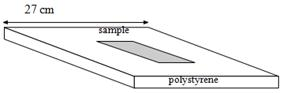Figure 3. Diagram of an embedded sample.

This method is used if the sample under consideration is small. In order to be able to use samples with sections smaller than standard, and to determine their conductivity or diffusivity, they have to be placed in a parallelepipedic polystyrene matrix, since the box is designed to receive samples of size 27 cm x 27 cm. The sample is placed as show in Figure 3.

In steady state, a part of the flow passes through the sample and another through the matrix of polystyrene. Considering that the flow remains unidirectional, its preservation for a given medium is written as follows: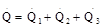With:: Electrical power emitted by the resistance (film) shaped by.: Lateral losses toward the room atmosphere (expressed by:).: Thermal power through the polystyrene, expressed by: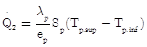where ep is the thickness of the polystyrene, Sp, also called measurement area, is its area useful.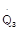: Thermal power running through the sample and expressed as: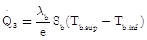.

The index b refers to the box.In steady state, we express: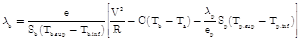(1.4)

Therefore, we conducted an experimental verification of the results given by this method and another on a sample of size 27 x 27 of same nature.

4. Introduction and Use of the Experimental Results Related to the Thermo Physical Characteristics

In the following paragraphs, we try to accurately follow the behavior of thermo physical parameters as a function of water content. These parameters are essentially the thermal conductivity (l) and thermal diffusivity (a).We will study the influence of moisture on these two parameters.

4.1. Change as a Function of Water Content

4.1.1. Thermal Conductivity

Conductivity is the measurement that characterizes the ability of the materials to let heat through. We know that the more a material is a conductor of heat, the higher it conductivity is. The choice made on the dirt as a reference material is justified by the fact that among the construction materials used in Chad, it plays an important role. For the tests, we varied the water content of the soil in each composition, starting from a sample containing a certain amount of water that is gradually dried until completely dry. The initial water content is obtained from an addition of a certain amount of water to the sample previously conditioned for 48 hours in a closed environment with less variable room temperature and humidity in order to allow the water to be distributed the most evenly possible. We simultaneously report the results of two selected soils.

a)  Earth only

The results obtained are shown in the following figure.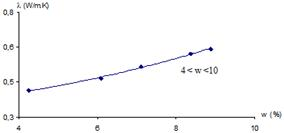Figure 4. Variation of conductivity l according to the water content w: dirt only (N'Djamena).

b)  Earth and straw

The results obtained are shown in Figure 5. The moisture content w was calculated in relation to the mass of the completely dry sample obtained by baking it in an oven for 24 hours and at a temperature of 105 ° C.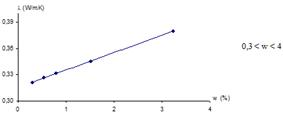Figure 5. Variation of conductivity as a function of water content: dirt and straw (N’Djamena).

Interpretation of results:

With regards to the results shown in the previous figures, we notice that the thermal conductivity increases with water content, regardless of the composition of the samples. Since the samples are porous materials, continuous membranes of liquid water with higher thermal conductivity than the dry air contained in the pores are formed in the capillary spaces, consequently resulting in an increase of the apparent thermal conductivity. In fact, KALBOUSSI  had shown in his work that, for light materials, thermal conductivity increases in a roughly parabolic function of density. Thermal conductivity of the samples can be estimated in relation with their density by connecting them through a degree 2 polynomial. And as the density and water content change linearly, we connected the points of the thermal conductivity of our measurements in relation with the water content by a degree 2 polynomial.

4.1.2. Thermal Diffusivity

Thermal diffusivity is related to a propagation speed of the thermal wave. In presenting the results, we followed the previously adopted plan in the case of thermal conductivity. The results given below are obtained from the formulations derived from the expressions of the model of the flash pulse on the sample , , .

a)  Earth only

The measurements yielded the following results summarized in Figure 6.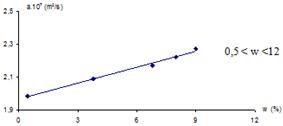Figure 6. Variation of the diffusivity as a function of water content: Earth only (N'Djamena).

b)  Earth and straw

In order to assess the amount of straw actually introduced, we can compare the densities of earth only and earth plus straw. For the form of use of dirt and straw combination, we obtained the following results: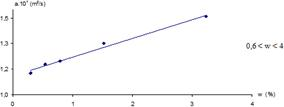Figure 7. Variation of the diffusivity as a function of water content: earth and straw (N'Djamena).

Interpretation of results: For all the measurements done, we found that diffusivity increases slightly with water content, which shows that when dirt is wet, it transmits heat more than when it is dry.

4.1.3. Mass-Related Heat

Here we present the values of specific heat taken from the measured values obtained previously. Indeed, having obtained the thermal conductivity and diffusivity by direct measurements, we deduced the specific heat by the relation Cp = l/ar.

a)  Earth only

The calculation gave us the following results for earth used alone: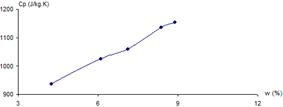Figure 8. Variation of mass-related heat as a function of water content:land only (N'Djamena).

b)  Earth and straw

For dirt mixed with straw, we obtained the results collected in Figure 9.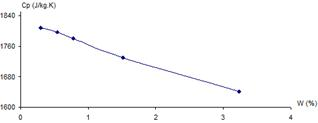Figure 9. Variation of mass-related heat as a function of water content: earth and straw (N'Djamena).

4.1.4. Thermal Resistance

In all the tables that follow, the values for thermal resistance are obtained using the formula: Rth = e/l where e is the thickness of the sample under consideration .

a)  Earth only

The calculation results of thermal resistance for earth alone without addition are shown in fig. 10.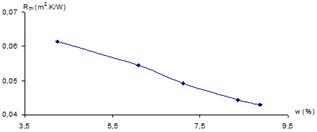Figure 10. Variation of thermal resistance as a function of water content: land only (N'Djamena).

b)  Earth and straw

The calculation results of thermal resistance for earth with added straw are shown in Fig.11.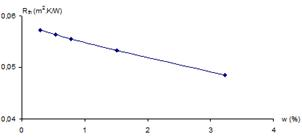Figure 11. Variation of thermal resistance as a function of water content: earth and straw (N'Djamena).

4.2. Change in Thermal Conductivity as a Function of Temperature

For the influence of temperature, we only studied the case of the thermal conductivity l we consider an important parameter of the out layer in the transfer of heat. For this, we varied the atmosphere of the measurement boxes by working on the supply voltage of the heating film. We performed the measurement of the parameter sought (l) each time the steady state was established.

According to the theory of gases, the temperature of an element of matter is proportional to the kinetic energy of microscopic particles that constitute it. Thus, the higher the temperature is, the faster its particles move. The extension of these results to the solid and liquid media shows that the pure electrolytic conductivity is a function of average temperature. The theoretical approach to the study of the apparent conductivity of a porous medium according to the average temperature may be conducted based on parallel series model , ,  . This approach as we have just shown for porous composite materials, would fit better for the case of our samples made of earth. As for porous medium that are opaque to radiation, the apparent thermal conductivity is given by: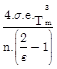(3.1)

ls =los (1 + bs Tm)                       (3.2)

lf =lof (1 + bfTm)                        (3.3)

q: Parallel conduction fraction

PT: total porosity.

ls: thermal conductivity of the solid (earth and straw).

lf: thermal conductivity of the fluid part.

bs: temperature coefficient of the solid medium.

bf: temperature coefficient of the fluid medium.

los: thermal conductivity of pure conduction at 0 °C, the solid part.

lof: thermal conductivity of pure conduction at 0 °C, the fluid part.

n: number of heat shields used in the model.

The combination of equations (3.1), (3.2) and (3.3) gives an expression of the form:

lapp = lc0 + lct + lr                        (3.4)

lc0: thermal conductivity of pure conduction at 0 °C

lct: term reflecting the change in conduction pure in relation with temperature

lr: thermal conductivity of internal radiation in the pores.

The amount lr is negligible as compared to lc and lct for the temperature range considered in our experiments (0 to 50 °C).

Thus theoretically, we obtain the following expression for the thermal conductivity:

lapp = A + B.Tm                          (3.5)

As A and B are constants, it can be assumed in first approximation that thermal conductivity is a linear function of the average temperature of the environment under consideration. In order to verify the theoretical reasoning outlined, we varied the voltage of the heating film. The variation of the supply voltage of the heating film increased the average temperature of the sample. Once the steady state was established, we proceeded to determine lwith varied the average temperature of materials in temperature ranges corresponding to the dry environment in the tropical zones. The results obtained are shown in the tables below. Then we plotted least-squares curve of fitness between the thermal conductivity and the average temperature of the sample.

a)  Dirt only sample

We present the results of measurements of thermal conductivity for the composition of earth alone without addition in relation with temperature in Figure 12.Figure 12. Variation of conductivity as a function of the average temperature: earth only (N'Djamena).

b)  Dirt and straw sample

We present the results of measurements of thermal conductivity for the soil with added straw as a function of temperature in Figure 13.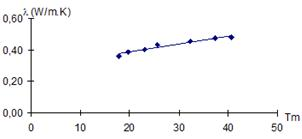Figure 13. Variation of conductivity as a function of the average temperature: earth and straw (N'Djamena).

Interpretation of results:

For each sample of the material studied, the curves obtained show that the conductivity varies linearly with the average temperature. In our case, this variation is very small and it is confirmed by the narrow range of temperatures considered [0 - 50 °C]. We relied on these temperatures because they are broadly representative of ambient temperatures in the Sahel.

5. Testing of Thermal Modeling and Comparison with Experimental Results

This part of our work is a study approach designed to present key information needed to assess the variation of the apparent thermal conductivity of materials previously studied as a function of water content.

5.1. Proposed Model

For modeling, ,  we assume that the materials studied in relation to the water content are tri-phasic porous materials: a gas, liquid and a solid phase by: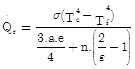(3.5)

For low temperature gradients Tc - Tf below 30 K it can be assumed that:

Tc4 -Tf4@ 4.Tm3. (Tc -Tf)                  (3.6)

With Tm=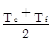, the average temperature of the environment.

Assuming that the gas is non-absorbent (a = 0) the density of heat flux exchanged by radiation within the material is: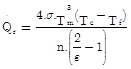(3.7)

Thus, thermal conductivity induced by radiative exchange and by analogy with the thermal conductivity is: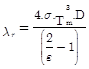(3.8)

With D =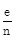, the average pore diameter.

Taking into account the experimental measuring conditions and the pore dimensions of the materials we studied, convection and radiation transfers are negligible.

To simplify the study, we adopt the model proposed by KRISCHER for two phases taking into account the above assumptions discussed above.

5.2. Validity of the Model

In order to calculate the thermal conductivity of the materials studied with the model we proposed, we had to experimentally determine two parameters, namely the thermal conductivity of the solid matrix lsand the volume fraction of the parallel phaseq. For us, the gas phase is the air the thermal conductivity of which we fix at 0.023 W/mK and the liquid phase is water with a conductivity estimated at 0.57 W/mK, assuming that on average, measurements were performed at 20 °C.

5.2.1. Determination of lsand q

In order to model the heat level of these materials, we had to search for the conductivity of the solid structure ls of each of them. The structure of solid or solid matrix material is the dry part of material, excluding the pores.

Thus, modeling requires knowledge of ls that corresponds to the conductivity of the material compressed up to the total disappearance of the pores, which is even more difficult to achieve as this does not happen in a vacuum and air is incompressible. However, knowledge of rs helps to obtain the conductivity of the solid structure by calculation. To determine rs we used the simple method described below. We had a scaled cylinder in which we poured a quantity of water with known mass me and volume Ve. By adding an amount of crushed material and measuring the mass mT and the volume VT as a whole, we obtained the mass m and volume V of the added material. Density of the solid matrix is given by the relation:

rs =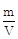(3.9)

With: m = mT - me and V = VT - Ve

Measurements of the density of different samples gave results that we recorded in Table 2.

Table 2. Values of the density of the solid structure of Samples.

 Name N’Djamena Measure earth only earth and straw mT (g) 82,531 90,747 VT (cm3) 76,000 82,000 me (g) 70,060 69,810 m (g) 15,471 20,937 V (cm3) 6,000 12,000 s (kg/m3) 2579 1745

After determining rs we made the determination of the porosity PT. The porosity of a sample is given by the relation: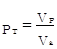(3.10)

with: VP, pores volume and Va the total apparent volume. The porous material consisting of solid and pores, this relationship can be expressed as: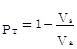(3.11)

Where Vs: the volume of the solid structure.

In representing the density of the solid structure by rs and the apparent density of the dry material by ra, we show that the above relation is marked as shown below: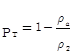(3.12)

We use this last expression (4.12) to determine the porosity of the materials studied. The calculations provided the results shown below in Table 3.

Table 3. Calculated values of the porosity of different samples.

 Name N’Djamena Measure earth only earth and straw a (kg/m3) 2068 1529 s (kg/m3) 2579 1745 PT 0,198 0,125

In order to determine ls we conducted two tests one of which on dry material and dry and material in saturated state in the other. In the dry state, the fluid phase was air, and in the saturated condition, the fluid phase was water. The apparent thermal conductivity depends on the thermal conductivity of the solid structure and the thermal conductivity of fluids of the phase or phase lf as well as on the degree of resistance on the part of the arrangement of phases. The distribution of each phase is random in the porous material.

It is believed that the porous material is replaced by a system of flat plates (Figure 6), each representing a phase. The plates are arranged in parallel, then in series so that the thermal conductivity reached by the model equals that of the material under consideration.

The thermal conductivity of a parallel configuration of volume fraction q is given by:

leff =l// = (1 - PT)ls + PTlf               (3.13)

And the configuration of a series of volume fraction (1 - q) is: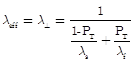(3.14)

When the porous body is dry, its thermal conductivity depends on the thermal conductivity of the solid structure ls and that of the thermal conductivity of the air filling the volume of the pores. And when it is saturated, its thermal conductivity depends on that of the water included in the volume of the pores and of ls. By applying the relationship 3.1, we will have the following systems of equation: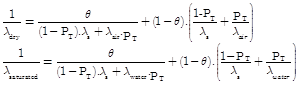(3.15)

Table 4. Calculated values of ls and q for the different samples.

 N’Djamena Measure Earth only Earthand straw ls (W/m.K) 0,63 0,59 q 0,88 0,75

To solve this system of nonlinear equations with two unknowns by the software Mathcad provided the results reported in Table 4.

5.2.2. Results of the Calculation of Thermal Conductivity of the Material from the Model

In order to calculate the apparent thermal conductivity of the materials studied from the model for given water content, we need to know the rate of water by any fraction of volume.

The rate of water volume se is the ratio between the volume of water contained in the material and the apparent volume. When the material is dry, the pores are empty and se equals zero. The value of se in any state is given by:

se =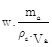(3.16)

With: w, water contents of the sample ma, and Va respectively the mass and the apparent volume of the sample and re the density of water.

From se, we deduce the rate of air volume in sa(sa = PT - se) for a given value of water content and we present the results by the study area as shown below. The following are samples made with the dirt of N'Djamena.

Table 5. Thermal conductivities Values calculated with the proposed model (N'Djamena).

 Land only Earth and straw w (%) se(%) sa(%) leff (W/m.K) w (%) se(%) sa(%) leff (W/m.K) 0,0 0,0 19,8 0,34 0,0 0,0 12,5 0,32 4,3 8,9 10,9 0,43 0,3 0,5 12,0 0,32 6,1 12,6 7,2 0,48 0,5 0,8 11,7 0,33 7,1 14,7 5,1 0,52 0,8 1,2 11,3 0,33 8,4 17,4 2,4 0,57 1,5 2,3 10,2 0,35 8,9 18,4 1,4 0,59 3,2 4,9 7,6 0,39

5.2.3. Comparison of Theoretical Models with Experimental Results

To compare experimental results with theoretical models presented in the previous section of this study, we present the variations of thermal conductivity calculated and experimentally determined as figures for this area.The results for samples made with earth taken from N'Djamena are presented in the following figures: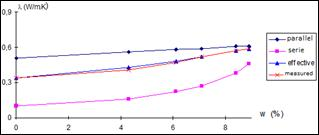Figure 14. Calculated and measured thermal conductivity variations: earth only (N'Djamena).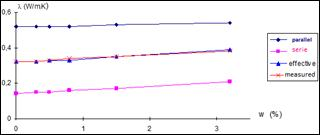Figure 15. Calculated and measured thermal conductivity variations: earthand straw (N'Djamena).

5.3. Conclusion

The comparison of the values of the thermal conductivity of the material with the model for given water content and the experimental results shows very good correspondence of results and justifies the validity of this model. Indeed, with the error margins of about 5% on the values of lmes the figures show that the curves of the proposed model have behaviors essentially identical to the experimental results for the materials studied. As for the values given by the serial and parallel theoretical models, they are situated on either side of the measured thermal conductivity values.

6. General Conclusion

In the work presented above, we relied on experimental results. We would like to complete this work by analyzing how a thermal study can be done in practice as well as in theory, by applying it to concrete cases in order to identify the common measures that could be used in other studies.

In practical terms, we found interesting to look deeply and physically into the properties characterizing the thermal behavior of an envelope between two different ambient environments such as thermal conductivity, thermal diffusivity, the thermal resistance and thermal capacity. Just as in our so-called Sahel countries, the most widely used material for construction of habitat is earth. We chose this material as a reference material even though in the mechanical point of view it is not a durable one. We presented the techniques for measuring conductivity and thermal diffusivity of the material earth. Different values of thermal conductivity, thermal diffusivity, thermal resistance and capacity of this material were presented and their variation as a function of parameters such as moisture content and temperature (for a single case). A model of distribution of the structure of earth was selected (parallel series). A comparison of the measured values with those calculated by the model used showed good agreement. As a result, we believe to contribute to a better definition of the thermal parameters of this material in order to help designers to more precisely evaluate the exchanges established between the external environment and within an enclosure made of earth.

References

1. S. GAYE. Contribution à l'étude de confort thermique en climat tropical: influence de la mouillure cutanée.Thèse de doctorat: UCAD. DAKAR (Sénégal). 1998.125p.
2. M. AHMAT-CHARFADINE. Etude expérimentale des propriétés thermo physiques des matériaux locaux du Tchad. Application au confort thermique de l’habitat. Thèse de Doctorat: Energétique: Université Claude Bernard Lyon 1: 06 Mars 2002 - 187p.
3. R. YEZOU. Contribution à l'étude des propriétés des matériaux de construction cohérentes et non cohérentes.-221p. Thèse: INSA Lyon (FRANCE): 1978.
4. S. ZEGADI. Etude théorique et expérimentale des transferts thermiques dans les milieux granulaires.- 195p. Thèse de Doctorat: Energétique: Université Claude Bernard Lyon 1: 22 Mai 1997; -97p.
5. S. BOUSAD., EZBAKHE, A. EL BAKKOUR, T. AJZOUL, A. EL BOUARDI.Etude thermique de la terre stabilisée au ciment utilisée en construction au Nord du Maroc. Revue Energies Renouvelables: Journées de Thermique, 2001 pp. 69-72.
6. A. DEGIOVANNI. Diffusivité et Méthode de Flash. Contribution à l'étude de la diffusivité thermique.Revue Générale de Thermique, 185, 1977 pp. 420-442.
7. AHMED ALI Ep. AIT KADI, S. Performances thermiques du matériau terre pour un habitat durable des régions arides et semi-arides: cas de Timimoune. Mémoire de Magistère: Université Mouloud Mammeri. TIZI-OUZOU (Algérie). 2012 - 109p.
8. R. SIEGEL, J. R. HOWELL. Thermal radiation heat transfer Mc GRAW HILL Book Company, N.Y 1972.
9. G. MENGUY, H. EZBAKHE, J. LEVEAU. Influence de la porosité sur les caractéristiques thermiques des matériaux de construction.First international Congress Held by RILEM of AFREM, vol.1, pp.269-270, Versailles (France). Septembre 1987.
10. A. KALBOUSSI. Transfert de chaleur en régime stationnaire et dynamique à travers les milieux poreux humides. - 238 p. Thèse de Doctorat.: Energétique: Université Claude Bernard Lyon 1: 1990; 40-90.
11. Mourtada, A. (1993). Thermique du bâtiment en climat chaud: Actes de l’Atelier sur "la maîtrise de l’énergie dans les bâtiments".Yaoundé, Cameroun: PRISME / IEPF / ADEME. - pp.32-51.
12. A. Mokhtari, K. Brahimi et R. Benziada, ‘Architecture et Confort Thermique dansles Zones Arides, Application au Cas de la Ville de Béchar’, Revue des Energies Renouvelables, Vol. 11, N°2, pp. 307 – 315, 2008.
13. Philippi I., Batsale J.C., Maillet D., Degiovanni A., Measurement of thermal diffusivity through processingof infrared images, Rev. Sci. Instrum. 66(1) 1995, 182-192.
14. Krapez JC, Spagnolo L., FrieB M., Maier H.P., Neuer, Measurement of in-plane diffusivity in nonhomogeneousslabs by applying flash thermography, International Journal of Thermal Sciences 43. (2004) 967-977.
15. A.W. Aregba, C.Pradere, J.-C. Batsale. Measurements thermo physical properties of materials by infrared thermography.2IE. Conférence Internationale: Eco matériaux de Construction. Du 10 au 12 Juin 2013 à Ouagadougou.180p.
16. V. K. Mathur. Composite materials from local resourcesConstruction and Building Materials. Volume 20, Issue 7, September 2006, Pages 470–477
17. Emmanuel Ouedraogo and al. Mechanical and Thermophysical Properties of Cement and/or Paper (Cellulose) Stabilized Compressed Clay Bricks. Journal of Materials and Engineering Structures 2 (2015) 68–76.
18. Mustapha BOUMHAOUT and al. Mesure de la conductivité thermique des matériaux de construction de différentes tailles par la méthode des boites. 3ème Congres de l'Association Marocaine de Thermique. Agadir (Maroc) 21-22 Avril 2014. p1-6.
19. K.GADRI & A. GUETTALA. Etude des caractéristiques physico-mécaniques des bétons de sable à base de fumée de silice.MATEC Web of Conferences. Volume 11, 2014. International Congress on Materials & Structural Stability.
20. Chao-Lung Hwang,Trong-Phuoc Huynh. Investigation into the use of unground rice husk ash to produce eco-friendly construction bricks.Construction and Building Materials.Volume 93, September 2015, Pages 335–341.

 Contents 1. 2. 2.1. 3. 3.1. 4. 4.1. 4.2. 5. 5.1. 5.2. 5.3. 6.
Article ToolsAbstractPDF(342K)